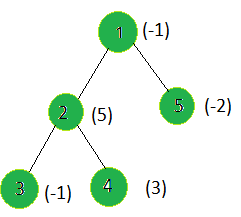GeeksforGeeks App
Open AppBrowser
Continue

# Find the root of the sub-tree whose weighted sum XOR with X is minimum

Given a tree, and the weights of all the nodes, the task is to find the root of the sub-tree whose weighted sum XOR with given integer X is minimum.
Examples:

Input:X = 15
Output:
Weight of sub-tree for parent 1 = ((-1) + (5) + (-2) + (-1) + (3)) XOR 15 = 4 XOR 15 = 11
Weight of sub-tree for parent 2 = ((5) + (-1) + (3)) XOR 15 = 7 XOR 15 = 8
Weight of sub-tree for parent 3 = -1 XOR 15 = -16
Weight of sub-tree for parent 4 = 3 XOR 15 = 12
Weight of sub-tree for parent 5 = -2 XOR 15 = -15
Node 3 gives the minimum sub-tree weighted sum XOR X.

Approach: Perform dfs on the tree, and for every node calculate the sub-tree weighted sum rooted at the current node then find the minimum (sum XOR X) value for a node.
Below is the implementation of the above approach:

## C++

 `// C++ implementation of the approach``#include ``using` `namespace` `std;` `int` `ans = 0, mini = INT_MAX;` `vector<``int``> graph;``vector<``int``> weight(100);` `// Function to perform dfs and update the tree``// such that every node's weight is the sum of``// the weights of all the nodes in the sub-tree``// of the current node including itself``void` `dfs(``int` `node, ``int` `parent)``{``    ``for` `(``int` `to : graph[node]) {``        ``if` `(to == parent)``            ``continue``;``        ``dfs(to, node);` `        ``// Calculating the weighted``        ``// sum of the subtree``        ``weight[node] += weight[to];``    ``}``}` `// Function to find the node``// having minimum sub-tree sum XOR x``void` `findMinX(``int` `n, ``int` `x)``{` `    ``// For every node``    ``for` `(``int` `i = 1; i <= n; i++) {` `        ``// If current node's weight XOR x``        ``// is minimum so far``        ``if` `(mini > (weight[i] ^ x)) {``            ``mini = (weight[i] ^ x);``            ``ans = i;``        ``}``    ``}``}` `// Driver code``int` `main()``{``    ``int` `x = 15;``    ``int` `n = 5;` `    ``// Weights of the node``    ``weight = -1;``    ``weight = 5;``    ``weight = -1;``    ``weight = 3;``    ``weight = -2;` `    ``// Edges of the tree``    ``graph.push_back(2);``    ``graph.push_back(3);``    ``graph.push_back(4);``    ``graph.push_back(5);` `    ``dfs(1, 1);``    ``findMinX(n, x);` `    ``cout << ans;` `    ``return` `0;``}`

## Java

 `// Java implementation of the approach``import` `java.util.*;` `class` `GFG``{``    ``static` `int` `ans = ``0``, mini = Integer.MAX_VALUE;` `    ``static` `Vector[] graph = ``new` `Vector[``100``];``    ``static` `Integer[] weight = ``new` `Integer[``100``];` `    ``// Function to perform dfs and update the tree``    ``// such that every node's weight is the sum of``    ``// the weights of all the nodes in the sub-tree``    ``// of the current node including itself``    ``static` `void` `dfs(``int` `node, ``int` `parent)``    ``{``        ``for` `(``int` `to : graph[node])``        ``{``            ``if` `(to == parent)``                ``continue``;``            ``dfs(to, node);` `            ``// Calculating the weighted``            ``// sum of the subtree``            ``weight[node] += weight[to];``        ``}``    ``}` `    ``// Function to find the node``    ``// having minimum sub-tree sum XOR x``    ``static` `void` `findMinX(``int` `n, ``int` `x)``    ``{` `        ``// For every node``        ``for` `(``int` `i = ``1``; i <= n; i++)``        ``{` `            ``// If current node's weight XOR x``            ``// is minimum so far``            ``if` `(mini > (weight[i] ^ x))``            ``{``                ``mini = (weight[i] ^ x);``                ``ans = i;``            ``}``        ``}``    ``}` `    ``// Driver code``    ``public` `static` `void` `main(String[] args)``    ``{``        ``int` `x = ``15``;``        ``int` `n = ``5``;``        ``for` `(``int` `i = ``0``; i < ``100``; i++)``            ``graph[i] = ``new` `Vector();``        ` `        ``// Weights of the node``        ``weight[``1``] = -``1``;``        ``weight[``2``] = ``5``;``        ``weight[``3``] = -``1``;``        ``weight[``4``] = ``3``;``        ``weight[``5``] = -``2``;` `        ``// Edges of the tree``        ``graph[``1``].add(``2``);``        ``graph[``2``].add(``3``);``        ``graph[``2``].add(``4``);``        ``graph[``1``].add(``5``);` `        ``dfs(``1``, ``1``);``        ``findMinX(n, x);` `        ``System.out.print(ans);``    ``}``}` `// This code is contributed by Rajput-Ji`

## Python3

 `# Python3 implementation of the approach``ans ``=` `0``mini ``=` `2``*``*``32` `graph ``=` `[[] ``for` `i ``in` `range``(``100``)]``weight ``=` `[``0``] ``*` `100` `# Function to perform dfs and update the tree``# such that every node's weight is the sum of``# the weights of all the nodes in the sub-tree``# of the current node including itself``def` `dfs(node, parent):``    ``global` `ans, mini, graph, weight, x``    ``for` `to ``in` `graph[node]:``        ``if` `(to ``=``=` `parent):``            ``continue``        ``dfs(to, node)``        ` `        ``# Calculating the weighted``        ``# sum of the subtree``        ``weight[node] ``+``=` `weight[to]``        ` `# Function to find the node``# having minimum sub-tree sum XOR x``def` `findMinX(n, x):``    ``global` `ans, mini,graph,weight``    ` `    ``# For every node``    ``for` `i ``in` `range``(``1``, n ``+` `1``):``        ` `        ``# If current node's weight XOR x``        ``# is minimum so far``        ``if` `(mini > (weight[i] ^ x)):``            ``mini ``=` `(weight[i] ^ x)``            ``ans ``=` `i``            ` `# Driver code``x ``=` `15``n ``=` `5` `# Weights of the node``weight[``1``] ``=` `-``1``weight[``2``] ``=` `5``weight[``3``] ``=` `-``1``weight[``4``] ``=` `3``weight[``5``] ``=` `-``2` `# Edges of the tree``graph[``1``].append(``2``)``graph[``2``].append(``3``)``graph[``2``].append(``4``)``graph[``1``].append(``5``)` `dfs(``1``, ``1``)``findMinX(n, x)` `print``(ans)` `# This code is contributed by SHUBHAMSINGH10`

## C#

 `// C# implementation of the approach``using` `System;``using` `System.Collections.Generic;` `class` `GFG``{``    ``static` `int` `ans = 0, mini = ``int``.MaxValue;` `    ``static` `List<``int``>[] graph = ``new` `List<``int``>;``    ``static` `int``[] weight = ``new` `int``;` `    ``// Function to perform dfs and update the tree``    ``// such that every node's weight is the sum of``    ``// the weights of all the nodes in the sub-tree``    ``// of the current node including itself``    ``static` `void` `dfs(``int` `node, ``int` `parent)``    ``{``        ``foreach` `(``int` `to ``in` `graph[node])``        ``{``            ``if` `(to == parent)``                ``continue``;``            ``dfs(to, node);` `            ``// Calculating the weighted``            ``// sum of the subtree``            ``weight[node] += weight[to];``        ``}``    ``}` `    ``// Function to find the node``    ``// having minimum sub-tree sum XOR x``    ``static` `void` `findMinX(``int` `n, ``int` `x)``    ``{` `        ``// For every node``        ``for` `(``int` `i = 1; i <= n; i++)``        ``{` `            ``// If current node's weight XOR x``            ``// is minimum so far``            ``if` `(mini > (weight[i] ^ x))``            ``{``                ``mini = (weight[i] ^ x);``                ``ans = i;``            ``}``        ``}``    ``}` `    ``// Driver code``    ``public` `static` `void` `Main(String[] args)``    ``{``        ``int` `x = 15;``        ``int` `n = 5;``        ``for` `(``int` `i = 0; i < 100; i++)``            ``graph[i] = ``new` `List<``int``>();``        ` `        ``// Weights of the node``        ``weight = -1;``        ``weight = 5;``        ``weight = -1;``        ``weight = 3;``        ``weight = -2;` `        ``// Edges of the tree``        ``graph.Add(2);``        ``graph.Add(3);``        ``graph.Add(4);``        ``graph.Add(5);` `        ``dfs(1, 1);``        ``findMinX(n, x);` `        ``Console.Write(ans);``    ``}``}` `// This code is contributed by PrinciRaj1992`

## Javascript

 ``

Output:

`3`

Complexity Analysis:

• Time Complexity : O(N).
In dfs, every node of the tree is processed once and hence the complexity due to the dfs is O(N) if there are total N nodes in the tree. Therefore, the time complexity is O(N).
• Auxiliary Space : O(n).
Recursion stack space can be up to O(n).

My Personal Notes arrow_drop_up